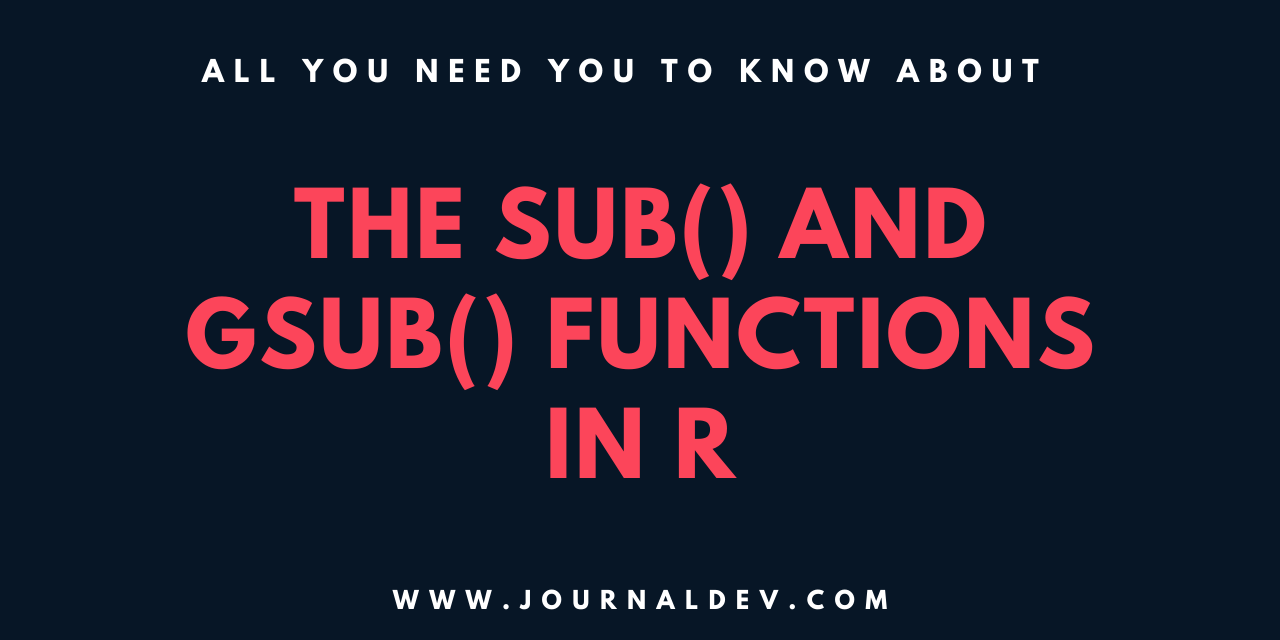# How To Use sub() and gsub() in R

Published on August 3, 2022 · Updated on December 6, 2022By Prajwal CN### Introduction

The `sub()` and `gsub()` functions in R will substitute the string or the characters in a vector or a data frame with a specific string. These functions are useful when performing changes on large data sets.

In this article, you will explore how to use `sub()` and `gsub()` functions in R.

## Prerequisites

To complete this tutorial, you will need:

## Syntax of `sub()` and `gsub()`

The basic syntax for `sub()` is:

``````sub(pattern, replacement, x)
``````

The basic syntax for `gsub()` is:

``````gsub(pattern, replacement, x)
``````

The syntax for `sub()` and `gsub()` requires a pattern, a replacement, and the vector or data frame:

• pattern: The pattern or the string which you want to be substituted.
• replacement: A input string to substitute the pattern string.
• x: A vector or a data frame to substitute the strings.

The pattern can also be in the form of a regular expression (regex).

Now that you are familiar with the syntax, you can move on to implementation.

## The `sub()` Function in R

The `sub()` function in R replaces the string in a vector or a data frame with the input or the specified string.

However, the limitation of the `sub()` function is that it only substitutes the first occurrence.

### 1. Using the `sub()` Function

In this example, learn how to substitute a string pattern with a replacement string with the `sub()` function.

``````# the input vector
df<-"R is an open-source programming language widely used for data analysis and statistical computing."

# the replacement
sub('R','The R language',df)
``````

Running this command generates the following output:

``````Output"The R language is an open-source programming language widely used for data analysis and statistical computing."
``````

The `sub()` function replaces the string `'R'` in the vector with the string `'The R language'`.

In this example, there was a single occurrence of pattern matching. Consider what happens if there are multiple occurrences of pattern matches.

``````# the input vector
df<-"In this tutorial, we will install R and show how to add packages from the official Comprehensive R Archive Network (CRAN)."

# the replacement
sub('R','The R language',df)
``````

Running this command generates the following output:

``````"In this tutorial, we will install The R language and show how to add packages from the official Comprehensive R Archive Network (CRAN)."
``````

In this example, you can observe that the `sub()` function replaced the first occurrence of the string `'R'` with `'The R language'`. But the next occurrence in the string remains the same.

### 2. Using the `sub()` Function with a Data Frame

The `sub()` function also works with data frames.

``````# creating a data frame
df<-data.frame(Creature=c('Starfish','Blue Crab','Bluefin Tuna','Blue Shark','Blue Whale'),Population=c(5,6,4,2,2))

# data frame
df
``````

This will create the following data frame:

``````      Creature Population
1     Starfish          5
2    Blue Crab          6
3 Bluefin Tuna          4
4   Blue Shark          2
5   Blue Whale          2
``````

Then replace the characters `'Blue'` with the characters `'Green'`:

``````# substituting the values
sub('Blue','Green',df)
``````

Running this command generates the following output:

``````Output"c(\"Starfish\", \"Green Crab\", \"Bluefin Tuna\", \"Blue Shark\", \"Blue Whale\")"
"c(5, 6, 4, 2, 2)"
``````

You can also specify a particular column to replace all the occurrences of `'Blue'` with `'Green'`:

``````# substituting the values
sub('Blue','Green',df\$Creature)
``````

Running this command generates the following output:

``````Output"Starfish"
"Green Crab"
"Greenfin Tuna"
"Green Shark"
"Green Whale"
``````

All instances of the characters `'Blue'` have been replaced with `'Green'`.

## The `gsub()` Function in R

The `gsub()` function in R is used for replacement operations. The function takes the input and substitutes it against the specified values.

Unlike the `sub()` function, `gsub()` applies a global substitution to all matches.

### 1. Using the `gsub()` Function

In this example, learn how to substitute a string pattern with a replacement string with the `gsub()` function.

``````# the input vector
df<-"In this tutorial, we will install R and show how to add packages from the official Comprehensive R Archive Network (CRAN)."
``````

This is data that has `'R'` written multiple times.

``````# substituting the values using gsub()
gsub('R','The R language',df)
``````
``````Output"In this tutorial, we will install The R language and show how to add packages from the official Comprehensive The R language Archive Network (CThe R languageAN)."
``````

All instances of ‘`R`’ have been replaced (including the instances in `"Comprehensive R Archive Network"` and `"CRAN"`). The `gsub()` function finds every word matching the parameter and replaces that with the input word or values.

### 2. Using the `gsub()` Function with Data Frames

The `gsub()` function also works with data frames.

``````# creating a data frame
df<-data.frame(Creature=c('Starfish','Blue Crab','Bluefin Tuna','Blue Shark','Blue Whale'),Population=c(5,6,4,2,2))
``````

Let’s prefix the values in the `Creature` column with `'Deep Sea '`:

``````# substituting the values
gsub('.*^','Deep Sea ',df\$Creature)
``````

Running this command generates the following output:

``````Output"Deep Sea Starfish"
"Deep Sea Blue Crab"
"Deep Sea Bluefin Tuna"
"Deep Sea Blue Shark"
"Deep Sea Blue Whale"
``````

In this example, the `gsub()` function uses the regular expression (regex): `.*^`. This is a pattern for the position at the start of the string.

## Conclusion

In this article, you explored how to use `sub()` and `gsub()` functions in R. These functions substitute the string or the characters in a vector or a data frame with a specific string. The `sub()` function applies for the first match. The `gsub()` function applies for all matches.

Continue your learning with How To Use `replace()` in R.

Thanks for learning with the DigitalOcean Community. Check out our offerings for compute, storage, networking, and managed databases.Prajwal CN

author#### Still looking for an answer?

Ask a questionSearch for more help

Click below to sign up and get \$200 of credit to try our products over 60 days!

### Popular Topics

##### Join the Tech Talk##### Hollie's Hub for Good

Working on improving health and education, reducing inequality, and spurring economic growth? We’d like to help.